Ex 4.1

Chapter 4 Class 10 Quadratic Equations
Serial order wise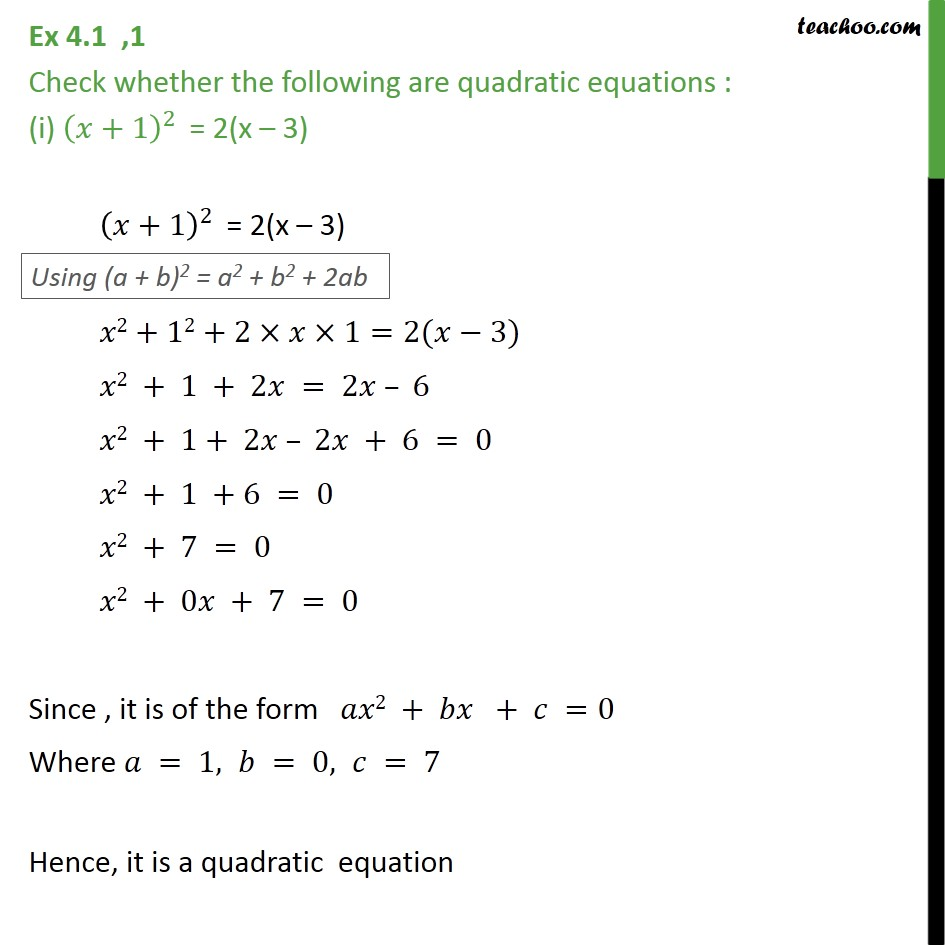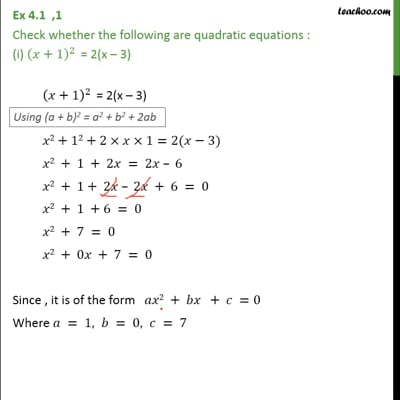This video is only available for Teachoo black users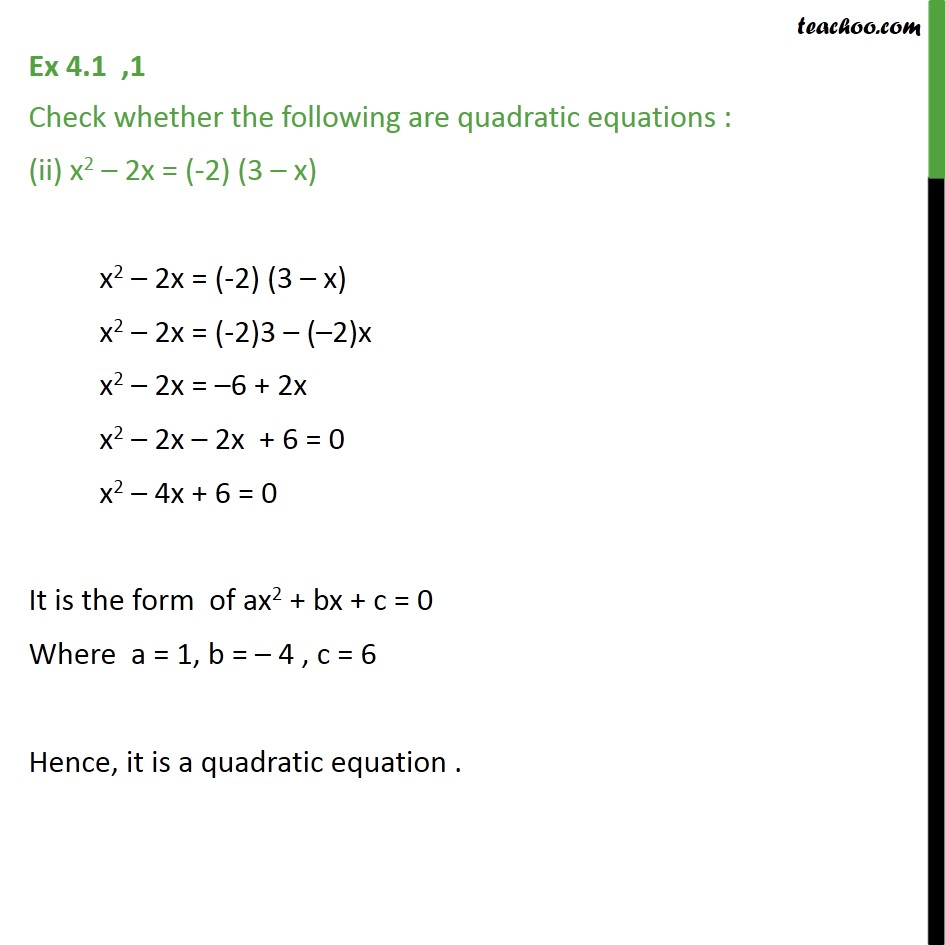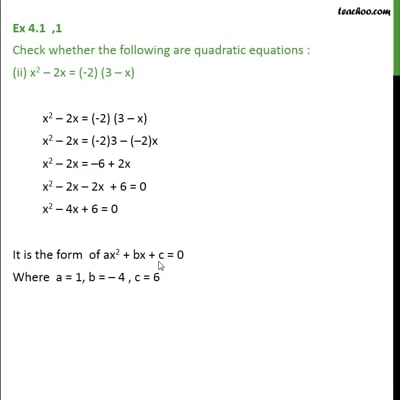This video is only available for Teachoo black users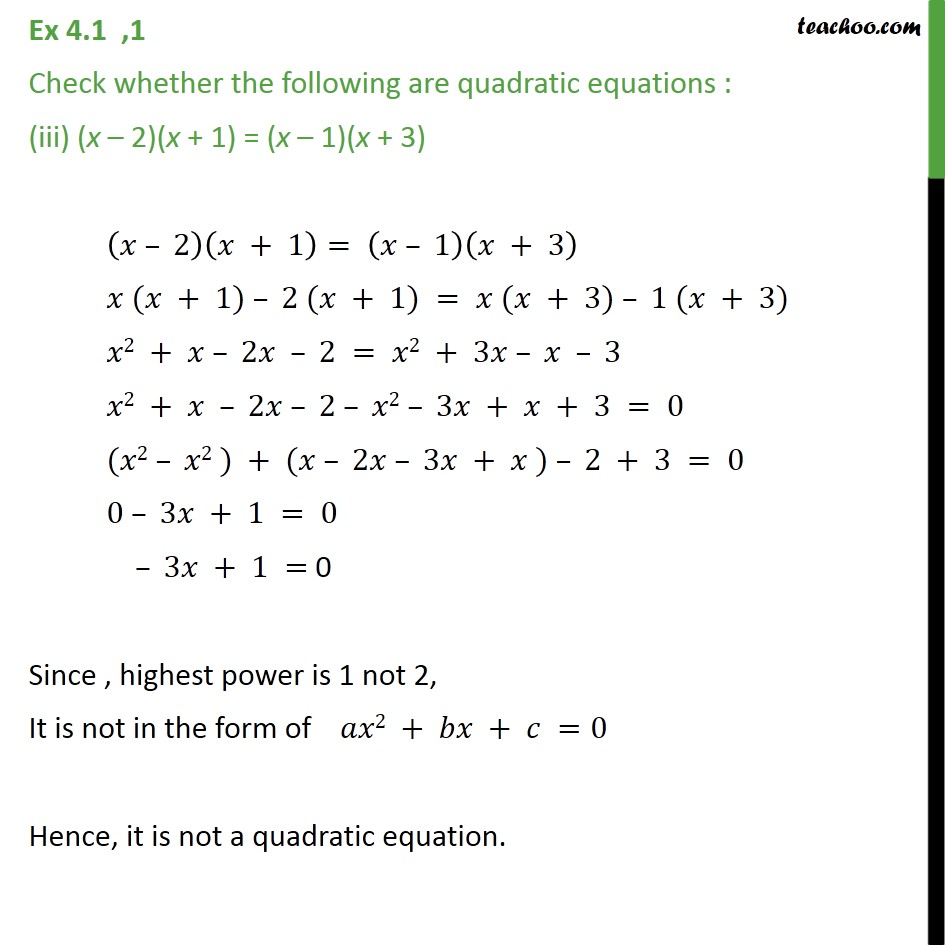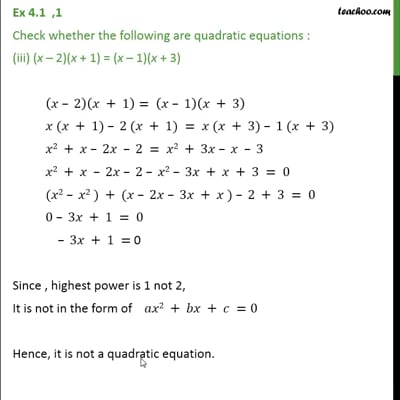This video is only available for Teachoo black users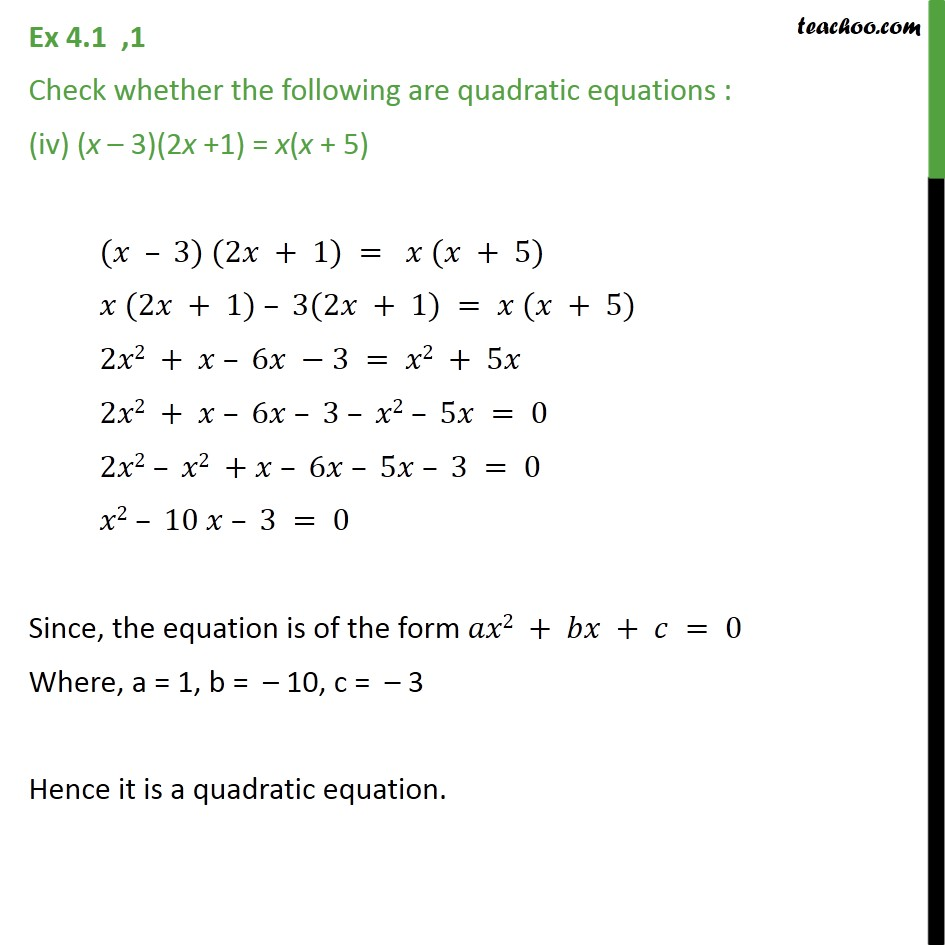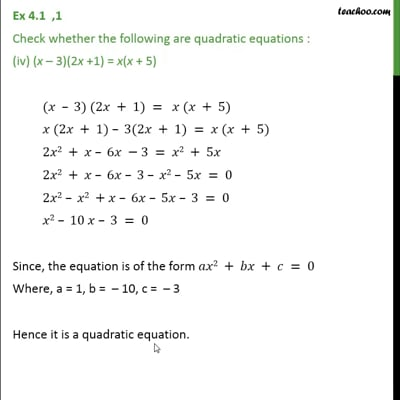This video is only available for Teachoo black users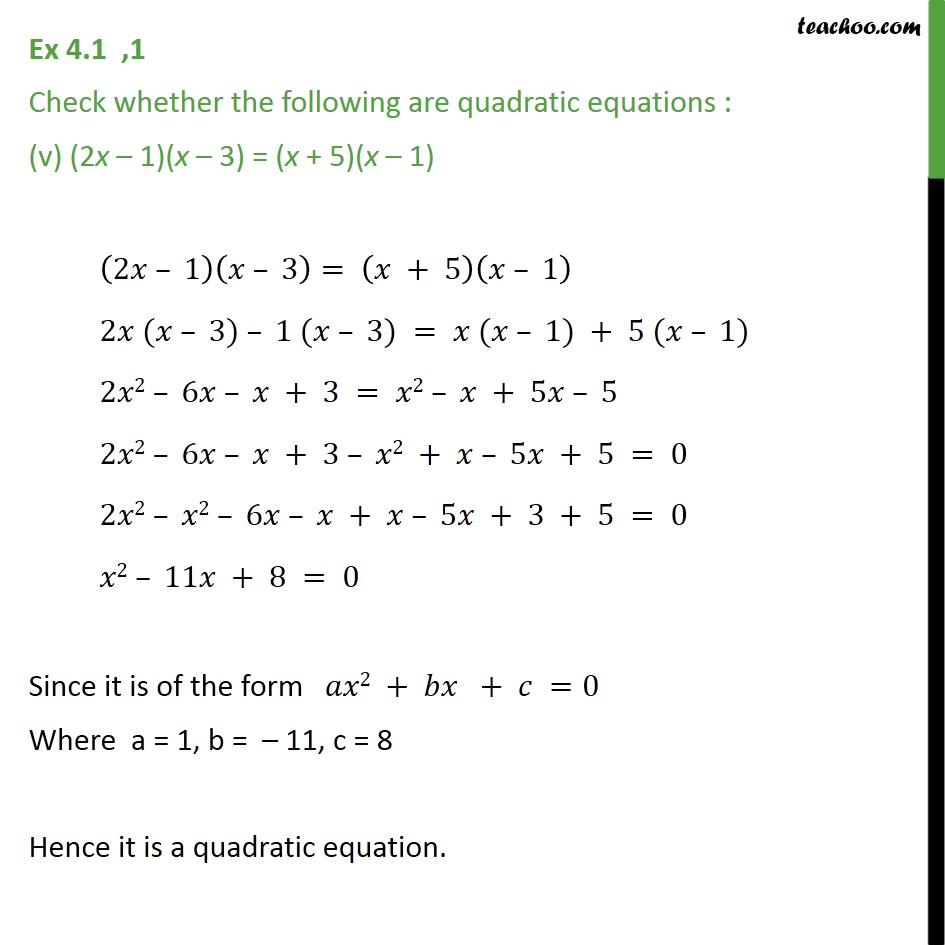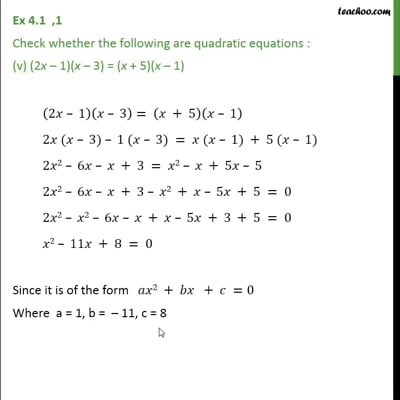This video is only available for Teachoo black users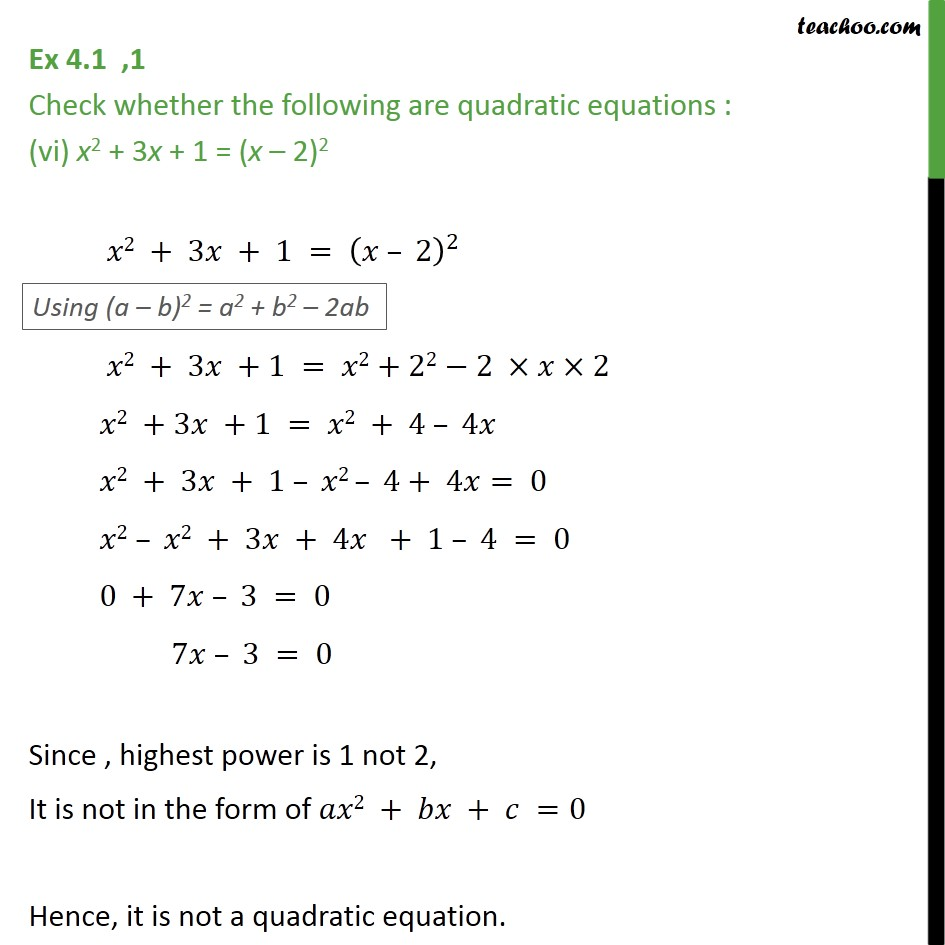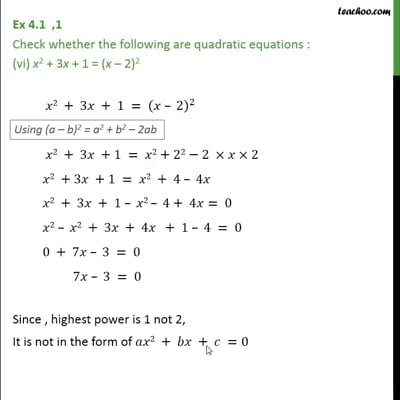This video is only available for Teachoo black users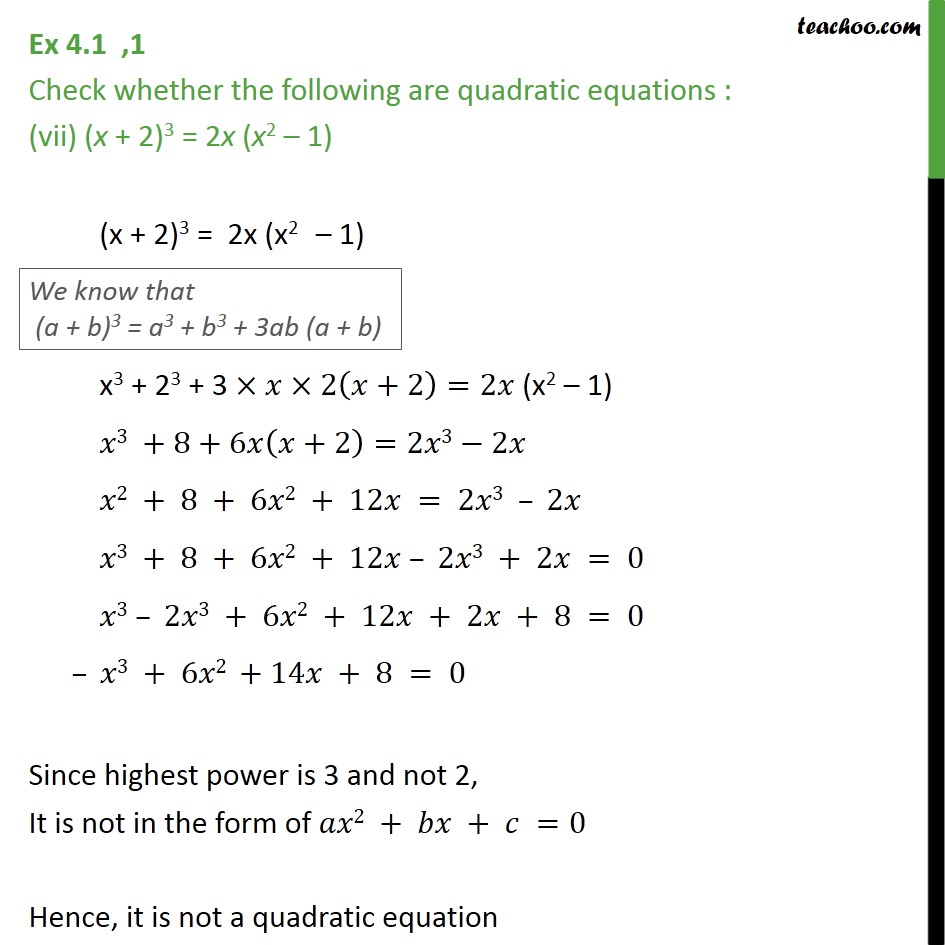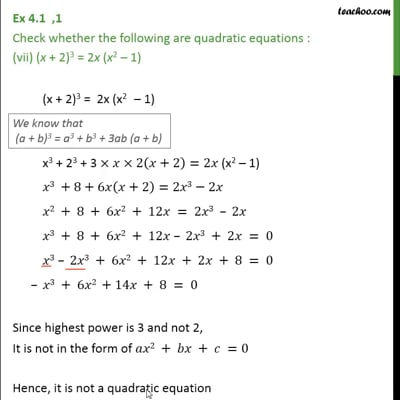This video is only available for Teachoo black users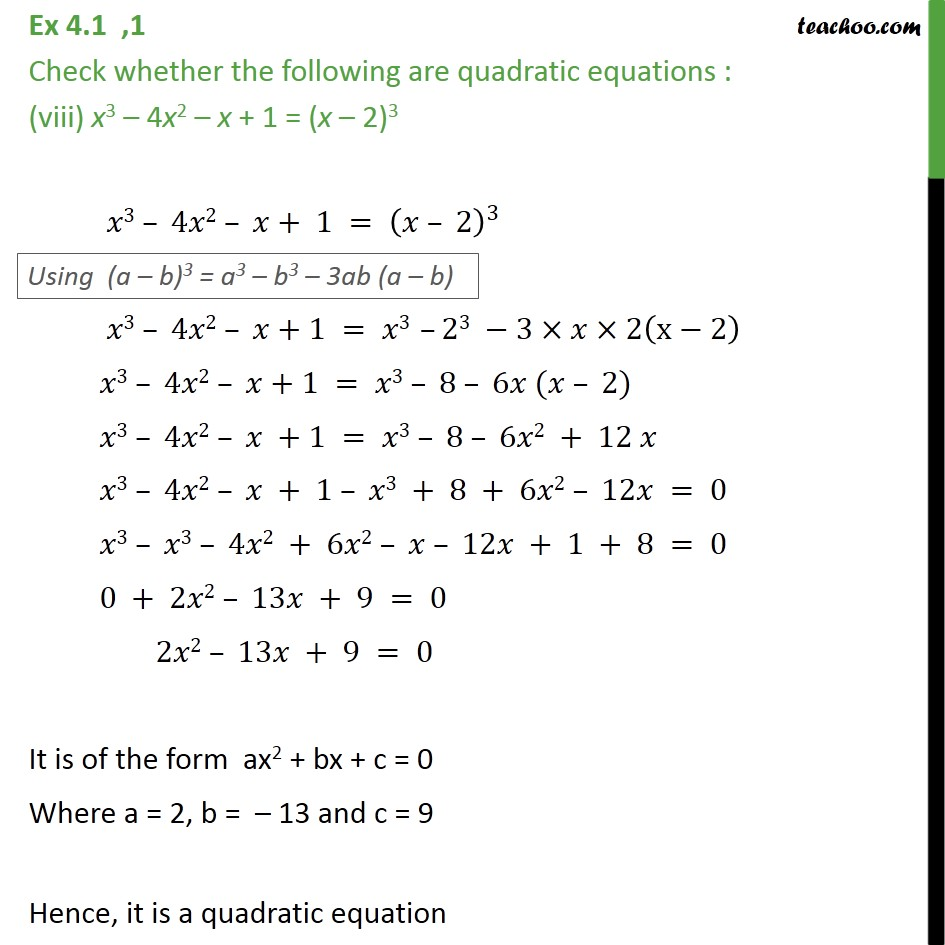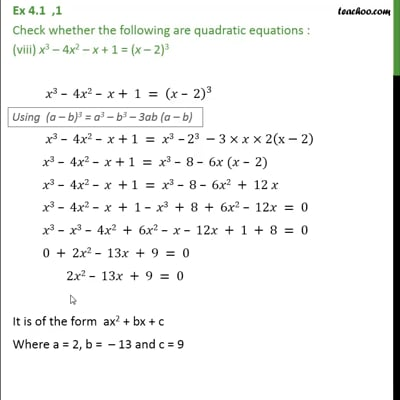This video is only available for Teachoo black users

Solve all your doubts with Teachoo Black (new monthly pack available now!)

### Transcript

Ex 4.1 ,1 Check whether the following are quadratic equations : (i) (𝑥+1)^2 = 2(x – 3) (𝑥+1)^2 = 2(x – 3) 𝑥2+12+2×𝑥×1=2(𝑥−3) 𝑥2 + 1 + 2𝑥 = 2𝑥 – 6 𝑥2 + 1+ 2𝑥 – 2𝑥 + 6 = 0 𝑥2 + 1 +6 = 0 𝑥2 + 7 = 0 𝑥2 + 0𝑥 + 7 = 0 Since , it is of the form 𝑎𝑥2 + 𝑏𝑥 + 𝑐 =0 Where 𝑎 = 1, 𝑏 = 0, 𝑐 = 7 Hence, it is a quadratic equation Ex 4.1 ,1 Check whether the following are quadratic equations : (ii) x2 – 2x = (-2) (3 – x) x2 – 2x = (-2) (3 – x) x2 – 2x = (-2)3 – (–2)x x2 – 2x = –6 + 2x x2 – 2x – 2x + 6 = 0 x2 – 4x + 6 = 0 It is the form of ax2 + bx + c = 0 Where a = 1, b = – 4 , c = 6 Hence, it is a quadratic equation . Ex 4.1 ,1 Check whether the following are quadratic equations : (iii) (x – 2)(x + 1) = (x – 1)(x + 3) (𝑥 – 2)(𝑥 + 1)= (𝑥 – 1)(𝑥 + 3) 𝑥 (𝑥 + 1) – 2 (𝑥 + 1) = 𝑥 (𝑥 + 3) – 1 (𝑥 + 3) 𝑥2 + 𝑥 – 2𝑥 – 2 = 𝑥2 + 3𝑥 – 𝑥 – 3 𝑥2 + 𝑥 – 2𝑥 – 2 – 𝑥2 – 3𝑥 + 𝑥 + 3 = 0 (𝑥2 – 𝑥2 ) + (𝑥 – 2𝑥 – 3𝑥 + 𝑥 ) – 2 + 3 = 0 0 – 3𝑥 + 1 = 0 – 3𝑥 + 1 = 0 Since , highest power is 1 not 2, It is not in the form of 𝑎𝑥2 + 𝑏𝑥 + 𝑐 =0 Hence, it is not a quadratic equation. Ex 4.1 ,1 Check whether the following are quadratic equations : (iv) (x – 3)(2x +1) = x(x + 5) (𝑥 – 3) (2𝑥 + 1) = 𝑥 (𝑥 + 5) 𝑥 (2𝑥 + 1) – 3(2𝑥 + 1) = 𝑥 (𝑥 + 5) 2𝑥2 + 𝑥 – 6𝑥 −3 = 𝑥2 + 5𝑥 2𝑥2 + 𝑥 – 6𝑥 – 3 – 𝑥2 – 5𝑥 = 0 2𝑥2 – 𝑥2 +𝑥 – 6𝑥 – 5𝑥 – 3 = 0 𝑥2 – 10 𝑥 – 3 = 0 Since, the equation is of the form 𝑎𝑥2 + 𝑏𝑥 + 𝑐 = 0 Where, a = 1, b = – 10, c = – 3 Hence it is a quadratic equation. Ex 4.1 ,1 Check whether the following are quadratic equations : (v) (2x – 1)(x – 3) = (x + 5)(x – 1) (2𝑥 – 1)(𝑥 – 3)= (𝑥 + 5)(𝑥 – 1) 2𝑥 (𝑥 – 3) – 1 (𝑥 – 3) = 𝑥 (𝑥 – 1) + 5 (𝑥 – 1) 2𝑥2 – 6𝑥 – 𝑥 + 3 = 𝑥2 – 𝑥 + 5𝑥 – 5 2𝑥2 – 6𝑥 – 𝑥 + 3 – 𝑥2 + 𝑥 – 5𝑥 + 5 = 0 2𝑥2 – 𝑥2 – 6𝑥 – 𝑥 + 𝑥 – 5𝑥 + 3 + 5 = 0 𝑥2 – 11𝑥 + 8 = 0 Since it is of the form 𝑎𝑥2 + 𝑏𝑥 + 𝑐 =0 Where a = 1, b = – 11, c = 8 Hence it is a quadratic equation. Ex 4.1 ,1 Check whether the following are quadratic equations : (vi) x2 + 3x + 1 = (x – 2)2 𝑥2 + 3𝑥 + 1 = (𝑥 – 2)^2 𝑥2 + 3𝑥 +1 = 𝑥2+22−2 ×𝑥×2 𝑥2 +3𝑥 +1 = 𝑥2 + 4 – 4𝑥 𝑥2 + 3𝑥 + 1 – 𝑥2 – 4+ 4𝑥= 0 𝑥2 – 𝑥2 + 3𝑥 + 4𝑥 + 1 – 4 = 0 0 + 7𝑥 – 3 = 0 7𝑥 – 3 = 0 Since , highest power is 1 not 2, It is not in the form of 𝑎𝑥2 + 𝑏𝑥 + 𝑐 =0 Hence, it is not a quadratic equation. Ex 4.1 ,1 Check whether the following are quadratic equations : (vii) (x + 2)3 = 2x (x2 – 1) (x + 2)3 = 2x (x2 – 1) x3 + 23 + 3 ×𝑥×2(𝑥+2)=2𝑥 (x2 – 1) 𝑥3 +8+6𝑥(𝑥+2)=2𝑥3−2𝑥 𝑥2 + 8 + 6𝑥2 + 12𝑥 = 2𝑥3 – 2𝑥 𝑥3 + 8 + 6𝑥2 + 12𝑥 – 2𝑥3 + 2𝑥 = 0 𝑥3 – 2𝑥3 + 6𝑥2 + 12𝑥 + 2𝑥 + 8 = 0 – 𝑥3 + 6𝑥2 +14𝑥 + 8 = 0 Since highest power is 3 and not 2, It is not in the form of 𝑎𝑥2 + 𝑏𝑥 + 𝑐 =0 Hence, it is not a quadratic equation Ex 4.1 ,1 Check whether the following are quadratic equations : (viii) x3 – 4x2 – x + 1 = (x – 2)3 𝑥3 – 4𝑥2 – 𝑥+ 1 = (𝑥 – 2)^3 𝑥3 – 4𝑥2 – 𝑥+1 = 𝑥3 –23 −3×𝑥×2(x−2) 𝑥3 – 4𝑥2 – 𝑥+1 = 𝑥3 – 8 – 6𝑥 (𝑥 – 2) 𝑥3 – 4𝑥2 – 𝑥 +1 = 𝑥3 – 8 – 6𝑥2 + 12 𝑥 𝑥3 – 4𝑥2 – 𝑥 + 1 – 𝑥3 + 8 + 6𝑥2 – 12𝑥 = 0 𝑥3 – 𝑥3 – 4𝑥2 + 6𝑥2 – 𝑥 – 12𝑥 + 1 + 8 = 0 0 + 2𝑥2 – 13𝑥 + 9 = 0 2𝑥2 – 13𝑥 + 9 = 0 It is of the form ax2 + bx + c = 0 Where a = 2, b = – 13 and c = 9 Hence, it is a quadratic equation